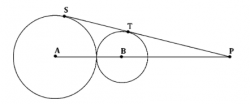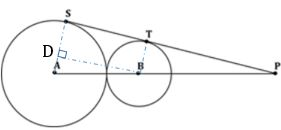GMAT Question of the Day: Daily via email | Daily via Instagram New to GMAT Club? Watch this Video

 It is currently 23 Jan 2020, 19:32### GMAT Club Daily Prep

#### Thank you for using the timer - this advanced tool can estimate your performance and suggest more practice questions. We have subscribed you to Daily Prep Questions via email.

Customized
for You

we will pick new questions that match your level based on your Timer History

Track

every week, we’ll send you an estimated GMAT score based on your performance

Practice
Pays

we will pick new questions that match your level based on your Timer History

#### Not interested in getting valuable practice questions and articles delivered to your email? No problem, unsubscribe here.# Common Mistakes in Geometry Questions - Exercise Question #4

Author Message
TAGS:

### Hide Tags

e-GMAT RepresentativeV
Joined: 04 Jan 2015
Posts: 3219
Common Mistakes in Geometry Questions - Exercise Question #4  [#permalink]

### Show Tags

3
4000:00

Difficulty:95% (hard)

Question Stats:29% (02:05) correct71% (02:11) wrongbased on 495 sessions

### HideShow timer Statistics

Common Mistakes in Geometry Questions - Exercise Question #4

A bigger circle (with center A) and a smaller circle (with center B) are touching each other externally. PT and PS are the tangents drawn to these circles from an external point (as shown in the figure). What is the length of ST?(1) The radii of the bigger and the smaller circles are 9 cm and 4 cm respectively
(2) PB = 52/5 cm

A. Statement (1) ALONE is sufficient, but statement (2) alone is not sufficient.

B. Statement (2) ALONE is sufficient, but statement (1) alone is not sufficient.

C. BOTH statements TOGETHER are sufficient, but NEITHER statement ALONE is sufficient.

D. EACH statement ALONE is sufficient.

E. Statements (1) and (2) TOGETHER are NOT sufficient.

Common Mistakes in Geometry are explained in detail in the following post:

Common Mistakes in Geometry Questions

Detailed solution will be posted soon._________________

Originally posted by EgmatQuantExpert on 22 Nov 2016, 05:33.
Last edited by EgmatQuantExpert on 02 Dec 2016, 09:17, edited 2 times in total.
e-GMAT RepresentativeV
Joined: 04 Jan 2015
Posts: 3219
Re: Common Mistakes in Geometry Questions - Exercise Question #4  [#permalink]

### Show Tags

8
9
Note: This questions is related to the article on Common Errors in Geometry

Kindly go through the article once, before solving the question or going through the solution.

Official Solution

Steps 1 & 2: Understand Question and Draw Inferences

Given that PT is a tangent to the small circle and PS is a tangent to the big circle.
ΔPTB and ΔPSA are right angled at T and S respectively.
We need to find the length of ST.Step 3: Analyze Statement 1

(1) The radii of the bigger and the smaller circles are 9 cm and 4 cm respectively.
If we drop a perpendicular BD on side AS, we get:

BDST is a rectangle (since all angles of this quadrilateral are right angles).
Therefore, since opposite sides of a rectangle are equal, SD = BT = 4 cm
This means, AD = 9 – 4 = 5cm
In right triangle ADB, by applying Pythagoras Theorem, we get:
B$$D^2$$ + A$$D^2$$ = A$$B^2$$

That is, B$$D^2$$ = (9+4$$)^2$$ – (5$$)^2$$

B$$D^2$$ = (13+5)(13-5)

Therefore, BD = 12

Since opposite sides of a rectangle are equal, ST = BD = 12 cm
Since we have been able to determine a unique length of ST, Statement (1) is sufficient.

Step 4: Analyze Statement 2

(2) PB = 52/5 cm

In right triangle BTP, we know the length of only one side: BP.
In order to find the lengths of the other sides of this triangle, we need one more piece of information – either one of the two unknown angles, or one of the two unknown sides.
Since we don’t have this information, we will not be able to find the lengths of the unknown sides of triangle BTP.
Due to a similar reasoning, we will not be able to find the length of the sides of triangle ASP.
So, we will not have enough information to find the length of ST.

Therefore statement 2 is not sufficient to arrive at a unique answer.

Since we got the answer from the first statement, Correct Answer: A

Thanks,
Saquib
Quant Expert
e-GMAT_________________

Originally posted by EgmatQuantExpert on 22 Nov 2016, 11:11.
Last edited by EgmatQuantExpert on 01 Dec 2016, 01:58, edited 1 time in total.
##### General Discussion
e-GMAT RepresentativeV
Joined: 04 Jan 2015
Posts: 3219
Re: Common Mistakes in Geometry Questions - Exercise Question #4  [#permalink]

### Show Tags

1
Hey Everyone,

The official solution has been posted. Kindly go through it and if you have any doubts feel free to post your query.Thanks,
Saquib
Quant Expert
e-GMAT
_________________
InternB
Joined: 13 Dec 2017
Posts: 7
Location: India
Schools: ISB '20 (II)
GMAT 1: 710 Q47 V40
GPA: 3.9
Common Mistakes in Geometry Questions - Exercise Question #4  [#permalink]

### Show Tags

Hello,

I have a slight problem with your explanation as to why option 2 is wrong.
The question states that we have the value of PB as 52/5. You have mentioned that we do not have the value of BT, but BT is the radius of the circle and is equal to 4. Using pythagoras theorem, we can find the value of PT.

Similarly, in the bigger triangle, we have the value of PA as 52/5 + 4 + 9, and the value of AS as 9, and applying pythagoras theorem, we can find the value of PS.

Subtracting the value of PS by PT, we can find the value of ST.

Therefore, I believe that the answer should be D, please let me know if there is something faulty in my deduction regarding statement 2.

Regards.
InternJoined: 30 Aug 2017
Posts: 1
Re: Common Mistakes in Geometry Questions - Exercise Question #4  [#permalink]

### Show Tags

3
When you consider the second statement, you are not supposed to consider what is stated in the statement 1. In that case you won't know the radius of both the smaller and the bigger circle so statement 2 will remain insufficient only.Hence,answer A is right.

Posted from my mobile device
GMATH TeacherP
Status: GMATH founder
Joined: 12 Oct 2010
Posts: 935
Re: Common Mistakes in Geometry Questions - Exercise Question #4  [#permalink]

### Show Tags

3
EgmatQuantExpert wrote:
A bigger circle (with center A) and a smaller circle (with center B) are touching each other externally. PT and PS are the tangents drawn to these circles from an external point (as shown in the figure). What is the length of ST?(1) The radii of the bigger and the smaller circles are 9 cm and 4 cm respectively
(2) PB = 52/5 cm

$$? = ST$$

(1) Sufficient. Please follow the arguments below looking at the image attached.

$$\Delta PTB\,\, \cong \,\,\,\Delta PSA\,\,\,\,\,\, \Rightarrow \,\,\,\,\,\left\{ \begin{gathered} \,\frac{4}{9} = \,\frac{{4 + {\text{aux}}}}{{9 + 4 + 4 + {\text{aux}}}}\,\,\,\,\, \Rightarrow \,\,\,\,\,\,{\text{aux}}\,\,\,{\text{unique}}\,\,\,\,\,\mathop \Rightarrow \limits^{\left( * \right)} \,\,\,\,\,PT\,\,\,{\text{unique}} \hfill \\ \,\frac{9}{4} = \frac{{ST + PT}}{{PT}}\,\,\,\,\,\mathop \Rightarrow \limits^{PT\,\,{\text{unique}}} \,\,\,\,?\,\, = \,\,ST\,\,{\text{unique}}\,\,\,\,\, \Rightarrow \,\,\,\,\,\,{\text{SUFF}}. \hfill \\ \end{gathered} \right.$$

$$\left( * \right)\,\,\,\Delta PTB\,\,\,\left\{ \begin{gathered} TB = 4 \hfill \\ \left( {{\text{4}}\,{\text{ + }}\,{\text{aux}}} \right)\,\,{\text{unique}} \hfill \\ \end{gathered} \right.\,\,\,\,\mathop \Rightarrow \limits^{{\text{Pythagoras}}} \,\,\,\,\,PT\,\,\,\,{\text{unique}}$$

(2) Insufficient. We present the GEOMETRIC BIFURCATION in the image attached.

This solution follows the notations and rationale taught in the GMATH method.

Regards,
Fabio.
Attachments13_Set18_7t.gif [ 61.78 KiB | Viewed 3783 times ]

_________________
Fabio Skilnik :: GMATH method creator (Math for the GMAT)
Our high-level "quant" preparation starts here: https://gmath.net
InternB
Joined: 02 Apr 2013
Posts: 4
Re: Common Mistakes in Geometry Questions - Exercise Question #4  [#permalink]

### Show Tags

Dear all, I have a question : how do we know that P, T and S are all on the same line ? The text just says PT and PS are tangents, but according to the position of P, we could have tangents that are not aligned no ?

The text doesn't says it. Is it just based on the figure ? and the sentence " as shown in the figure" ?
Math ExpertV
Joined: 02 Sep 2009
Posts: 60627
Re: Common Mistakes in Geometry Questions - Exercise Question #4  [#permalink]

### Show Tags

EricD28 wrote:
Dear all, I have a question : how do we know that P, T and S are all on the same line ? The text just says PT and PS are tangents, but according to the position of P, we could have tangents that are not aligned no ?

The text doesn't says it. Is it just based on the figure ? and the sentence " as shown in the figure" ?

Problem Solving
Figures: All figures accompanying problem solving questions are intended to provide information useful in solving the problems. Figures are drawn as accurately as possible. Exceptions will be clearly noted. Lines shown as straight are straight, and lines that appear jagged are also straight. he positions of points, angles, regions, etc., exist in the order shown, and angle measures are greater than zero. All figures lie in a plane unless otherwise indicated.

Data Sufficiency:
Figures:
• Figures conform to the information given in the question, but will not necessarily conform to the additional information given in statements (1) and (2).
• Lines shown as straight are straight, and lines that appear jagged are also straight.
• The positions of points, angles, regions, etc., exist in the order shown, and angle measures are greater than zero.
• All figures lie in a plane unless otherwise indicated.
_________________
InternB
Joined: 02 Apr 2013
Posts: 4
Re: Common Mistakes in Geometry Questions - Exercise Question #4  [#permalink]

### Show Tags

Thanks a lot for this quick reply !
ManagerB
Joined: 25 Nov 2017
Posts: 78
Location: India
GMAT 1: 590 Q47 V25
GMAT 2: 660 Q50 V29
GPA: 3.56
Re: Common Mistakes in Geometry Questions - Exercise Question #4  [#permalink]

### Show Tags

Triangle PAS and triangle PBT are similar , AP/PB=SA/TB=PS/PT => (AP/PB)-1=(SA/TB)-1=(PS/PT)-1 => AB/PB=5/4=ST/PT => PT/PB=ST/AB=5/4
@e-GMAT : Is the underlined step right ?
e-GMAT RepresentativeV
Joined: 04 Jan 2015
Posts: 3219
Re: Common Mistakes in Geometry Questions - Exercise Question #4  [#permalink]

### Show Tags

BARUAH wrote:
Triangle PAS and triangle PBT are similar , AP/PB=SA/TB=PS/PT => (AP/PB)-1=(SA/TB)-1=(PS/PT)-1 => AB/PB=5/4=ST/PT => PT/PB=ST/AB=5/4
@e-GMAT : Is the underlined step right ?

Hey BARUAH,
can you provide a bit more details about how you are getting the value of AB/PB = 5/4?
_________________
InternB
Joined: 30 Sep 2019
Posts: 31
Re: Common Mistakes in Geometry Questions - Exercise Question #4  [#permalink]

### Show Tags

Can we approach this question through the following method-
join SB . So, BTS will be a right-angled triangle.

as per the Pythagorian triplets only the combination of 3,4,5 is possible.
So thus, ST can be determined.

Bunuel VeritasKarishma EgmatQuantExpert daagh chetan2u gmatbusters
Veritas Prep GMAT InstructorV
Joined: 16 Oct 2010
Posts: 10008
Location: Pune, India
Common Mistakes in Geometry Questions - Exercise Question #4  [#permalink]

### Show Tags

breatheanddoit wrote:
Can we approach this question through the following method-
join SB . So, BTS will be a right-angled triangle.

as per the Pythagorian triplets only the combination of 3,4,5 is possible.
So thus, ST can be determined.

Bunuel VeritasKarishma EgmatQuantExpert daagh chetan2u gmatbusters

Knowing one side of a right triangle is not enough to give the other two sides. Note that pythagorean triplets give only integral value triplets. A right angled triangle can have sides
4, 5, sqrt(41)
or 4, 7, sqrt(65)
or 4, 1, sqrt(17)
and many more...
_________________
Karishma
Veritas Prep GMAT InstructorCommon Mistakes in Geometry Questions - Exercise Question #4   [#permalink] 07 Nov 2019, 22:40
Display posts from previous: Sort by

# Common Mistakes in Geometry Questions - Exercise Question #4